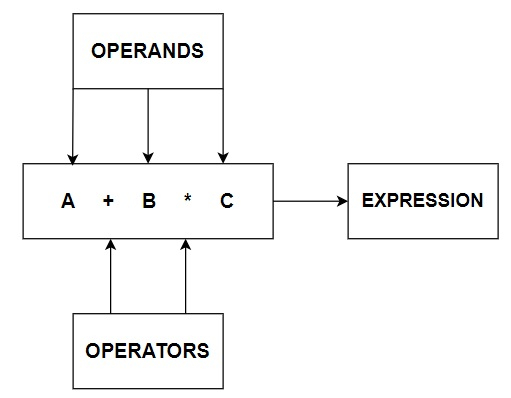# What are the relational operators in Java?

## OPERATORS

In computer programming we often need to perform some arithmetical or logical operations. It is then that we need operators to pitch in and perform these tasks. An Operator is basically a symbol or token, which performs arithmetical or logical operations and gives us meaningful result. The values involved in the operation are called Operands.

Here is a basic Pictorial representation of Operators.Now, let us discuss the types of Operators available.

### TYPES OF OPERATORS

There are three types of Operators in Java which are −

• Arithmetical Operators

• Relational Operators

• Logical Operators

## Relational OPERATORS

These operators are used to show the relationship among the operands. Relational operators compare the values of the variables and results in terms of ‘True’ or ‘False’ (i.e. 0 or 1).

The different types of relational operators are given below −

### Less than (<)

#### Example

This operator is used to verify if one operand is less than the other. For example, if a = 10 and b = 5, then a < b will return False, but b < a will return True. Here is an example code.

import java.util.*;
public class LessThanOperator{
public static void main(String[] args){
Scanner in = new Scanner(System.in);
System.out.println("Enter 1st element : ");
int a = in.nextInt();
System.out.println("Enter 2nd element : ");
int b = in.nextInt();
if (a < b) {  // Checking if a is less than b or not
System.out.println("Smallest Element is : "+ a);
} else {
System.out.println("Smallest Element is : "+ b);
}
}
}


#### Output

Enter 1st element :
10
Enter 2nd element :
5
Smallest Element is : 5


### Greater than (>)

#### Example

This operator is used to verify if one operand is greater than the other. For example, if a = 10 and b = 5, then a > b will return True, but b > a will return False. Here is an example code.

import java.util.*;
public class GreaterThanOperator{
public static void main(String[] args){
Scanner in = new Scanner(System.in);
System.out.println("Enter 1st element : ");
int a = in.nextInt();
System.out.println("Enter 2nd element : ");
int b = in.nextInt();
if (a > b){  // Checking if a is greater than b or not
System.out.println("Largest Element is : "+ a);
} else {
System.out.println("Largest Element is : "+ b);
}
}
}


#### Output

Enter 1st element :
10
Enter 2nd element :
5
Largest Element is : 10


### Less than or Equal to (<=)

This operator is used to verify if one operand is either less than or equal to the other. For example, if a = 10 and b = 5, then a <= b will return False, but b <= a will return True. Here is an example code.

#### Example

import java.util.*;
public class LessThanOrEqualToOperator{
public static void main(String[] args){
Scanner in = new Scanner(System.in);
System.out.println("Enter 1st element : ");
int a = in.nextInt();
System.out.println("Enter 2nd element : ");
int b = in.nextInt();
if (a <= b) {  // Checking if a is less than b or equal to b
System.out.println("Smallest Element is : "+ a);
} else {
System.out.println("Smallest Element is : "+ b);
}
}
}


#### Output

Enter 1st element :
10
Enter 2nd element :
5
Smallest Element is : 5


### Greater than or Equal to(>=)

#### Example

This operator is used to verify if one operand is either greater than or equal to the other. For example, if a = 10 and b = 5, then a >= b will return True, but b >= a will return False. Here is an example code.

import java.util.*;
public class GreaterThanOrEqualToOperator{
public static void main(String[] args){
Scanner in = new Scanner(System.in);
System.out.println("Enter 1st element : ");
int a = in.nextInt();
System.out.println("Enter 2nd element : ");
int b = in.nextInt();
if (a >= b){  // Checking if a is greater than b or equal to b
System.out.println("Largest Element is : "+ a);
} else {
System.out.println("Largest Element is : "+ b);
}
}
}


#### Output

Enter 1st element :
10
Enter 2nd element :
5
Largest Element is : 10


### Equal to (==)

This operator is used to verify if one operand is equal to the other. For example, if a = 10 and b = 5, then a == b will return False, but if a = 10 and b = 10, then a == b will return True. Here is an example code.

#### Example

import java.util.*;
public class EqualToOperator{
public static void main(String[] args){
Scanner in = new Scanner(System.in);
System.out.println("Enter 1st element : ");
int a = in.nextInt();
System.out.println("Enter 2nd element : ");
int b = in.nextInt();
if (a == b){  // Checking if a is equal to b
System.out.println("Equal”);
} else {
System.out.println("Not Equal”);
}
}
}


#### Output

Enter 1st element :
10
Enter 2nd element :
10
Equal


### Not Equal to (!=)

#### Example

This operator is used to verify if one operand is not equal to the other. For example, if a = 10 and b = 5, then a != b will return True, but if a = 10 and b = 10, then a != b will return False. Here is an example code.

import java.util.*;
public class NotEqualToOperator{
public static void main(String[] args){
Scanner in = new Scanner(System.in);
System.out.println("Enter 1st element : ");
int a = in.nextInt();
System.out.println("Enter 2nd element : ");
int b = in.nextInt();
if (a != b) {  // Checking if a is not equal to b
System.out.println("Not equal”);
} else {
System.out.println("Equal”);
}
}
}


#### Output

Enter 1st element :
10
Enter 2nd element :
5
Not equal